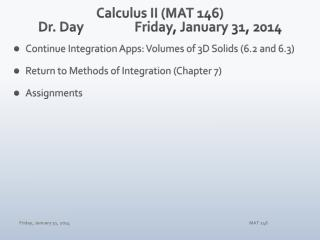Download PresentationCalculus II (MAT 146) Dr. Day Friday, January 31, 2014

# Calculus II (MAT 146) Dr. Day Friday, January 31, 2014 - PowerPoint PPT PresentationDownload Presentation## Calculus II (MAT 146) Dr. Day Friday, January 31, 2014

- - - - - - - - - - - - - - - - - - - - - - - - - - - E N D - - - - - - - - - - - - - - - - - - - - - - - - - - -
##### Presentation Transcript

1. Calculus II (MAT 146)Dr. Day Friday, January 31, 2014 • Continue Integration Apps: Volumes of 3D Solids (6.2 and 6.3) • Return to Methods of Integration (Chapter 7) • Assignments MAT 146

2. Volumes of Solids of Revolution (6.2 & 6.3) • Dynamic Illustration #1 (discs) • Dynamic Illustration #2 (washer) • Dynamic Illustration #3 (shell) • Dynamic Illustration #4 (cross section I) (cross section II) MAT 146

3. Consider the first-quadrant region R with borders y = sin(x) y = 0 and x = π/2 Sketch region R on the xy-plane. Calculate the exact area of R. Show evidence to support your solution. Set up, but do not calculate, a definite integral to represent the volume of the solid created when R is revolved around the y-axis. MAT 146

4. Consider the first-quadrant region R with borders y = sin(x) y = 0 and x = π/2 Sketch region R on the xy-plane. MAT 146

5. Consider the first-quadrant region R with borders y = sin(x) y = 0 and x = π/2 Calculate the exact area of R. Show evidence to support your solution. MAT 146

6. Consider the first-quadrant region R with borders y = sin(x) y = 0 and x = π/2 Set up, but do not calculate, a definite integral to represent the volume of the solid created when R is revolved around the y-axis. Shells: Washers: MAT 146

7. MAT 146

8. Undoing the Product Rule MAT 146

9. Integration by Parts • An Integral with a Product Integrand • Examples MAT 146

10. Integration by Parts • Key Component of Integrand’s Two Factors • For at least one factor, its derivative is “simpler” than the factor. • For at least one factor, its anti-derivative is no more complex than the factor. MAT 146

11. MAT 146

12. You Choosing U: A Decision Algorithm L: log functions I: inverse trig functions A: algebraic functions T: trig functions E: exponential functions MAT 146

13. Assignments • WebAssign: 6.2/6.3 Homework due Sunday at midnight • WebAssign: 7.1 Homework due Wednesday at midnight …and… WA Quiz #5: Available after class today! Test #1: Thurs & Fri, Feb 6 and Feb 7 MAT 146